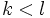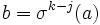# Cycle decomposition theorem for permutations

Jump to: navigation, search

## Statement

### Statement for a finite set

Any permutation on a finite set admits a cycle decomposition: it can be expressed as a product of a finite number of pairwise disjoint cycles.

Moreover, this cycle decomposition is unique.

## Importance

This result has several close analogues. One close analogue is for the general linear group, with the existence of a rational canonical form and a Jordan canonical form. A common framework that unifies results in both contexts is the framework of APS theory. In this framework, we say that the permutation IAPS admits unique class factorization.

Further information: permutation IAPS admits unique class factorization

## Proof

Let$S$ be the finite set and$\sigma:S \to S$ be a permutation.

Pick$a \in S$. Then, consider the sequence$a, \sigma(a), \sigma^2(a), \dots$. This sequence must eventually repeat, so there exist$k < l$ such that$\sigma^k(a) = \sigma^l(a)$, so by the definition of group action, we have$\sigma^{l-k}(a) = a$. Let$r$ be the smallest positive integer such that$\sigma^r(a) = a$. Then, we have a cycle:$(a, \sigma(a) ,\sigma^2(a), \dots, \sigma^{r-1}(a))$

Thus, every element of$S$ is part of a cycle. Moreover, any cycle containing$a$ has to be of the above form (up to a cyclic re-ordering).

It thus suffices to show that any two cycles obtained this way are either equal or disjoint. Suppose$a,b \in S$. Then, if the cycles of$a$ and$b$ are not disjoint, there exist$j,k$ such that$\sigma^j(b) = \sigma^k(a)$. This forces$b = \sigma^{k-j}(a)$, so$b$ is in the cycle of$a$. But then, starting the cycle at$b$, we see that the cycle of$b$ is the same as the cycle of$a$.